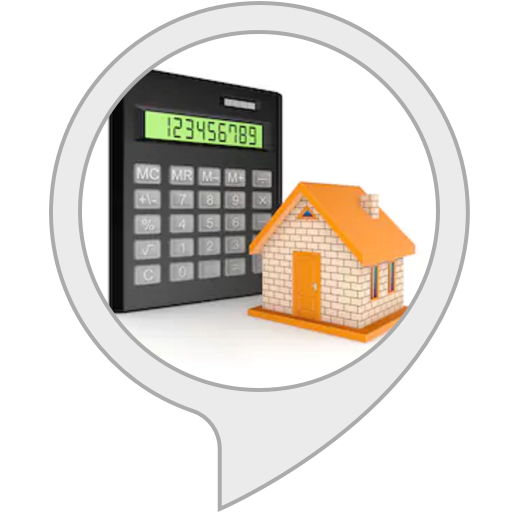# Loan Calculator

• Alexa, open loan calculator
• calculate total payment on four thousand for sixty months at two percentage
• calculate monthly payment on four thousand for sixty months at two percenta

Simple Alexa Skill to calculate loan via doing anything. No need to take your phone out of pocket or look for a calculator in drawer every time you need to calculate loan. Just ask Alexa to open loan calculator.

Example phrases:
1. calculate total payment on four thousand for sixty months at two percentage
2. total payment on four thousand for sixty months at two percentage
3. calculate monthly payment on four thousand for sixty months at two percentage
4. calculate yearly payment on four thousand for sixty months at two percentage